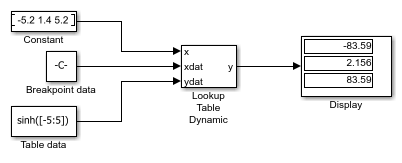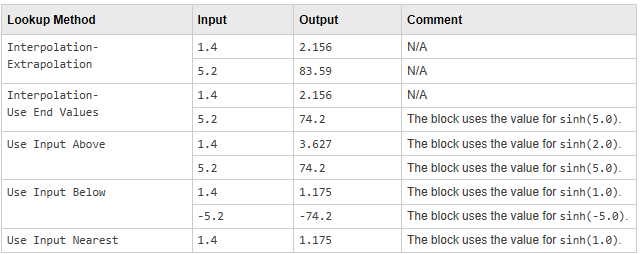# Approximating the sinh Function Using the Lookup Table Dynamic Block

This example shows how to use the Lookup Table Dynamic block to approximate the `sinh` function. The breakpoint data is given by the vector `[-5:5]` and the table data is given by the vector `sinh([-5:5])`. The input `x` is provided by the Constant block as a 1-by-3 vector containing values that are below, within, and above the breakpoint data values.

To see how each lookup method handles input values that are below, within, and above the breakpoint data values, change the value of the Lookup Method parameter on the Lookup Table Dynamic block.The Lookup Table Dynamic block outputs the following values when using the specified lookup methods and inputs.